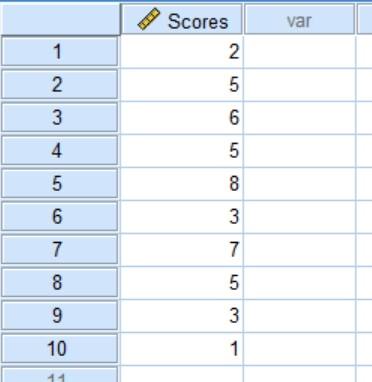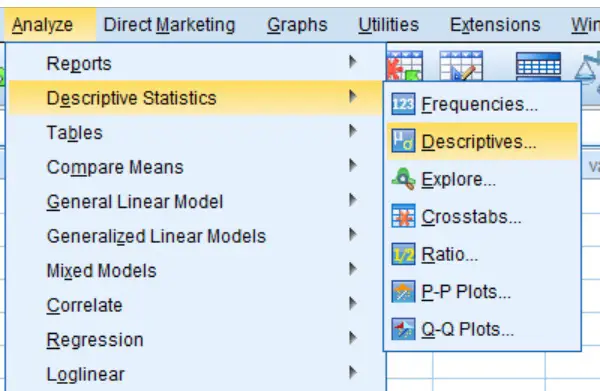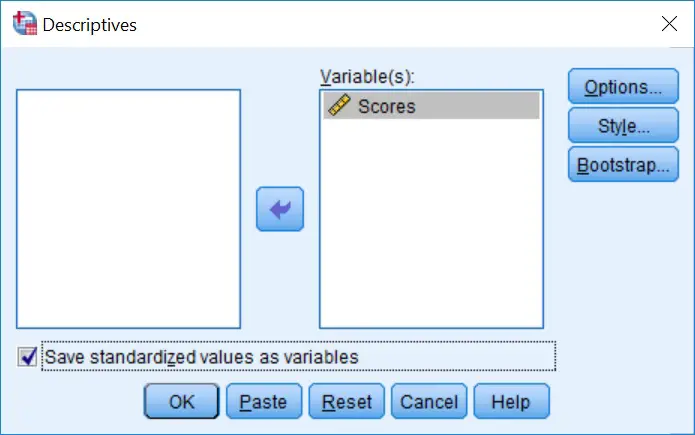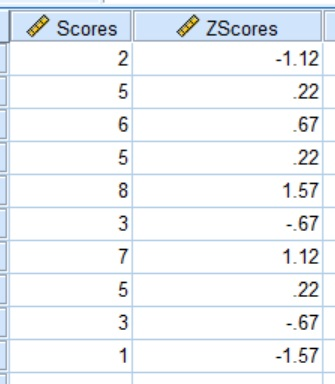How To Calculate Z-scores By Using SPSS

In this guide, I will explain how to calculate Z-scores by using SPSS, in three simple steps. The process is actually ridiculously easy, it’s just not very obvious within the SPSS interface on how to do it.

What is a Z-score?

A Z-score, also known as a standard score, represents the number of standard deviations (SDs) a data point is away from the average (mean) of the group. Z-scores, therefore, are a useful way of standardising values.

How to calculate Z-scores in SPSS

To do this, I will use an example, as mentioned previously. Within SPSS the data looks like this.Simply, it is just a list of 10 scores on a memory test.

1. To calculate Z-scores, firstly go to the Descriptives by going to Analyze > Descriptive Statistics > Descriptives... .2. Next, move the scores that need to be converted into the Variable(s) box to the right. Also, click the option to Save standardized values as variables. It is this simple tick box which enables the creation of Z-scores!3. Finally, click the OK button.

That’s all that is required!

If you now go to the Data View within SPSS, you should see a new variable has been created, which is named as the same variable as before with a Z prefix added. So, in the example, this is called Zscores. This is the Z-scores of the Scores variable we started with.So, that is how to use SPSS to create Z-scores very quickly.